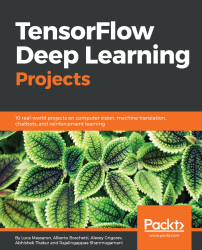# TensorFlow Deep Learning Projects5 (2 reviews total)
By Abhishek Thakur , Alberto Boschetti , Luca Massaron and 2 more
• \$33.99 eBook
• \$41.99 Print + eBook
• \$12.99 eBook + Subscription
What do you get with a Packt Subscription?

• Constantly updated with 100+ new titles each month
• Breadth and depth in over 1,000+ technologies
TensorFlow is one of the most popular frameworks used for machine learning and, more recently, deep learning. It provides a fast and efficient framework for training different kinds of deep learning models, with very high accuracy. This book is your guide to master deep learning with TensorFlow with the help of 10 real-world projects. TensorFlow Deep Learning Projects starts with setting up the right TensorFlow environment for deep learning. You'll learn how to train different types of deep learning models using TensorFlow, including Convolutional Neural Networks, Recurrent Neural Networks, LSTMs, and Generative Adversarial Networks. While doing this, you will build end-to-end deep learning solutions to tackle different real-world problems in image processing, recommendation systems, stock prediction, and building chatbots, to name a few. You will also develop systems that perform machine translation and use reinforcement learning techniques to play games. By the end of this book, you will have mastered all the concepts of deep learning and their implementation with TensorFlow, and will be able to build and train your own deep learning models with TensorFlow confidently.
Publication date:
March 2018
Publisher
Packt
Pages
320
ISBN
9781788398060

# Recognizing traffic signs using Convnets

As the first project of the book, we'll try to work on a simple model where deep learning performs very well: traffic sign recognition. Briefly, given a color image of a traffic sign, the model should recognize which signal it is. We will explore the following areas:

• How the dataset is composed
• Which deep network to use
• How to pre-process the images in the dataset
• How to train and make predictions with an eye on performance

# The dataset

Since we'll try to predict some traffic signs using their images, we will use a dataset built for the same purpose. Fortunately, researchers of Institute für Neuroinformatik, Germany, created a dataset containing almost 40,000 images, all different and related to 43 traffic signs. The dataset we will use is part of a competition named German Traffic Sign Recognition Benchmark (GTSRB), which attempted to score the performance of multiple models for the same goal. The dataset is pretty old—2011! But it looks like a nice and well-organized dataset to start our project from.

The dataset used in this project is freely available at http://benchmark.ini.rub.de/Dataset/GTSRB_Final_Training_Images.zip.

Before you start running the code, please download the file and unpack it in the same directory as the code. After decompressing the archive, you'll have a new folder, named GTSRB, containing the dataset.

The authors of the book would like to thank those who worked on the dataset and made it open source.

Let's now see some examples:

"Speed limit 20 km/h":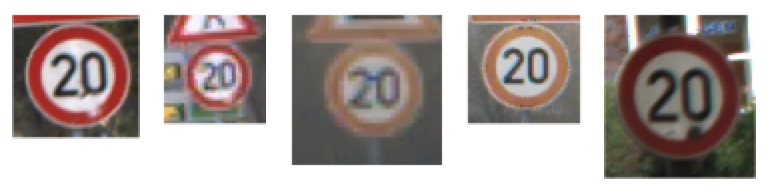"go straight or turn right":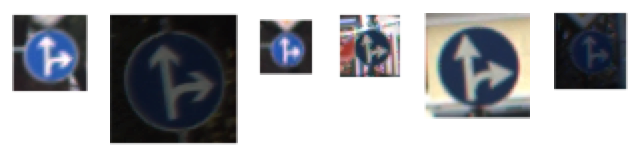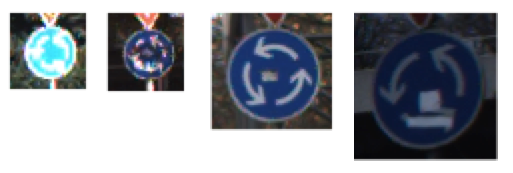As you can see, the signals don't have a uniform brightness (some are very dark and some others are very bright), they're different in size, the perspective is different, they have different backgrounds, and they may contain pieces of other traffic signs.

The dataset is organized in this way: all the images of the same label are inside the same folder. For example, inside the path GTSRB/Final_Training/Images/00040/, all the images have the same label, 40. For the images with another label, 5, open the folder GTSRB/Final_Training/Images/00005/. Note also that all the images are in PPM format, a lossless compression format for images with many open source decoders/encoders.

# The CNN network

For our project, we will use a pretty simple network with the following architecture: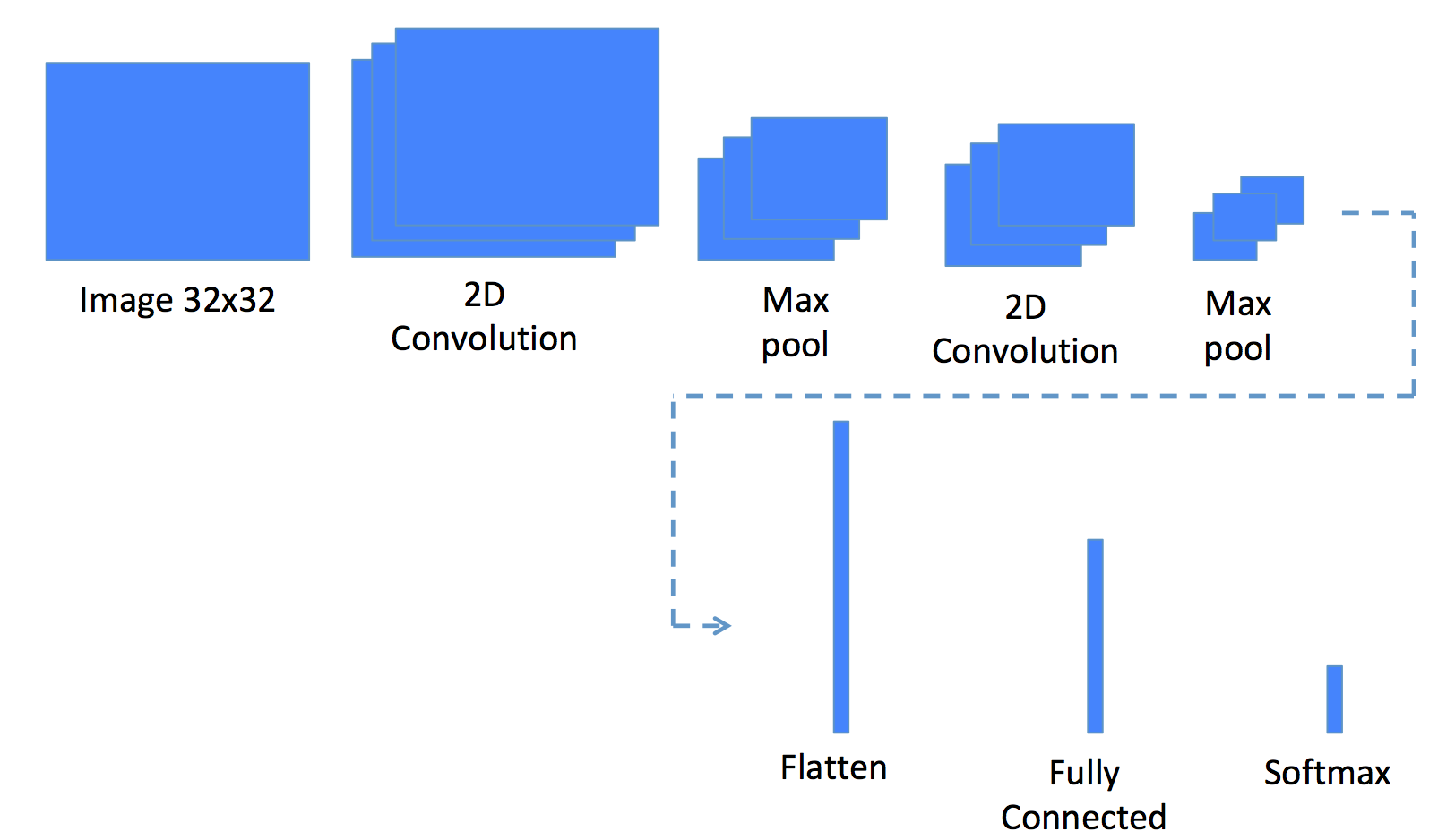In this architecture, we still have the choice of:

• The number of filters and kernel size in the 2D convolution
• The kernel size in the Max pool
• The number of units in the Fully Connected layer
• The batch size, optimization algorithm, learning step (eventually, its decay rate), activation function of each layer, and number of epochs

# Image preprocessing

The first operation of the model is reading the images and standardizing them. In fact, we cannot work with images of variable sizes; therefore, in this first step, we'll load the images and reshape them to a predefined size (32x32). Moreover, we will one-hot encode the labels in order to have a 43-dimensional array where only one element is enabled (it contains a 1), and we will convert the color space of the images from RGB to grayscale. By looking at the images, it seems obvious that the information we need is not contained in the color of the signal but in its shape and design.

Let's now open a Jupyter Notebook and place some code to do that. First of all, let's create some final variables containing the number of classes (43) and the size of the images after being resized:

`N_CLASSES = 43RESIZED_IMAGE = (32, 32)`

Next, we will write a function that reads all the images given in a path, resize them to a predefined shape, convert them to grayscale, and also one-hot encode the label. In order to do that, we'll use a named tuple named dataset:

`import matplotlib.pyplot as pltimport globfrom skimage.color import rgb2labfrom skimage.transform import resizefrom collections import namedtupleimport numpy as npnp.random.seed(101)%matplotlib inlineDataset = namedtuple('Dataset', ['X', 'y'])def to_tf_format(imgs):   return np.stack([img[:, :, np.newaxis] for img in imgs], axis=0).astype(np.float32)def read_dataset_ppm(rootpath, n_labels, resize_to):images = []labels = []for c in range(n_labels):   full_path = rootpath + '/' + format(c, '05d') + '/'   for img_name in glob.glob(full_path + "*.ppm"):         img = plt.imread(img_name).astype(np.float32)     img = rgb2lab(img / 255.0)[:,:,0]     if resize_to:       img = resize(img, resize_to, mode='reflect')         label = np.zeros((n_labels, ), dtype=np.float32)     label[c] = 1.0    images.append(img.astype(np.float32))     labels.append(label)return Dataset(X = to_tf_format(images).astype(np.float32),                 y = np.matrix(labels).astype(np.float32))dataset = read_dataset_ppm('GTSRB/Final_Training/Images', N_CLASSES, RESIZED_IMAGE)print(dataset.X.shape)print(dataset.y.shape)`

Thanks to the skimage module, the operation of reading, transforming, and resizing is pretty easy. In our implementation, we decided to convert the original color space (RGB) to lab, then retaining only the luminance component. Note that another good conversion here is YUV, where only the "Y" component should be retained as a grayscale image.

Running the preceding cell gives this:

`(39209, 32, 32, 1)(39209, 43)`

One note about the output format: the shape of the observation matrix X has four dimensions. The first indexes the observations (in this case, we have almost 40,000 of them); the other three dimensions contain the image (which is 32 pixel, by 32 pixels grayscale, that is, one-dimensional). This is the default shape when dealing with images in TensorFlow (see the code _tf_format function).

As for the label matrix, the rows index the observation, while the columns are the one-hot encoding of the label.

In order to have a better understanding of the observation matrix, let's print the feature vector of the first sample, together with its label:

`plt.imshow(dataset.X[0, :, :, :].reshape(RESIZED_IMAGE)) #sampleprint(dataset.y[0, :]) #label`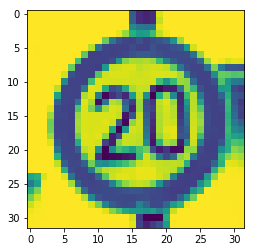`[[1. 0. 0. 0. 0. 0. 0. 0. 0. 0. 0. 0. 0. 0. 0. 0. 0. 0. 0. 0. 0. 0. 0. 0.0. 0. 0. 0. 0. 0. 0. 0. 0. 0. 0. 0. 0. 0. 0. 0. 0. 0.]]`

You can see that the image, that is, the feature vector, is 32x32. The label contains only one 1 in the first position.

Let's now print the last sample:

`plt.imshow(dataset.X[-1, :, :, :].reshape(RESIZED_IMAGE)) #sampleprint(dataset.y[-1, :]) #label`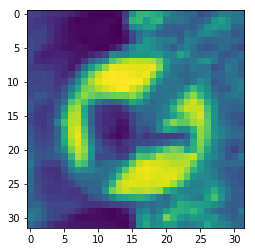`[[0. 0. 0. 0. 0. 0. 0. 0. 0. 0. 0. 0. 0. 0. 0. 0. 0. 0. 0. 0. 0. 0. 0. 0.0. 0. 0. 0. 0. 0. 0. 0. 0. 0. 0. 0. 0. 0. 0. 0. 0. 1.]]`

The feature vector size is the same (32x32), and the label vector contains one 1 in the last position.

These are the two pieces of information we need to create the model. Please, pay particular attention to the shapes, because they're crucial in deep learning while working with images; in contrast to classical machine learning observation matrices, here the X has four dimensions!

The last step of our preprocessing is the train/test split. We want to train our model on a subset of the dataset, and then measure the performance on the leftover samples, that is, the test set. To do so, let's use the function provided by sklearn:

`from sklearn.model_selection import train_test_splitidx_train, idx_test = train_test_split(range(dataset.X.shape), test_size=0.25, random_state=101)X_train = dataset.X[idx_train, :, :, :]X_test = dataset.X[idx_test, :, :, :]y_train = dataset.y[idx_train, :]y_test = dataset.y[idx_test, :]print(X_train.shape)print(y_train.shape)print(X_test.shape)print(y_test.shape)`

In this example, we'll use 75% of the samples in the dataset for training and the remaining 25% for testing. In fact, here's the output of the previous code:

`(29406, 32, 32, 1)(29406, 43)(9803, 32, 32, 1)(9803, 43)`

# Train the model and make predictions

The first thing to have is a function to create minibatches of training data. In fact, at each training iteration, we'd need to insert a minibatch of samples extracted from the training set. Here, we'll build a function that takes the observations, labels, and batch size as arguments and returns a minibatch generator. Furthermore, to introduce some variability in the training data, let's add another argument to the function, the possibility to shuffle the data to have different minibatches of data for each generator. Having different minibatches of data in each generator will force the model to learn the in-out connection and not memorize the sequence:

`def minibatcher(X, y, batch_size, shuffle):assert X.shape == y.shapen_samples = X.shapeif shuffle:   idx = np.random.permutation(n_samples)else:   idx = list(range(n_samples))for k in range(int(np.ceil(n_samples/batch_size))):   from_idx = k*batch_size   to_idx = (k+1)*batch_size   yield X[idx[from_idx:to_idx], :, :, :], y[idx[from_idx:to_idx], :]`

To test this function, let's print the shapes of minibatches while imposing batch_size=10000:

`for mb in minibatcher(X_train, y_train, 10000, True):print(mb.shape, mb.shape)`

That prints the following:

`(10000, 32, 32, 1) (10000, 43)(10000, 32, 32, 1) (10000, 43)(9406, 32, 32, 1) (9406, 43)`

Unsurprisingly, the 29,406 samples in the training set are split into two minibatches of 10,000 elements, with the last one of 9406 elements. Of course, there are the same number of elements in the label matrix too.

It's now time to build the model, finally! Let's first build the blocks that will compose the network. We can start creating the fully connected layer with a variable number of units (it's an argument), without activation. We've decided to use Xavier initialization for the coefficients (weights) and 0-initialization for the biases to have the layer centered and scaled properly. The output is simply the multiplication of the input tensor by the weights, plus the bias. Please take a look at the dimensionality of the weights, which is defined dynamically, and therefore can be used anywhere in the network:

`import tensorflow as tfdef fc_no_activation_layer(in_tensors, n_units):w = tf.get_variable('fc_W',   [in_tensors.get_shape(), n_units],   tf.float32,   tf.contrib.layers.xavier_initializer())b = tf.get_variable('fc_B',   [n_units, ],   tf.float32,   tf.constant_initializer(0.0))return tf.matmul(in_tensors, w) + b`

Let's now create the fully connected layer with activation; specifically, here we will use the leaky ReLU. As you can see, we can build this function using the previous one:

`def fc_layer(in_tensors, n_units):return tf.nn.leaky_relu(fc_no_activation_layer(in_tensors, n_units))`

Finally, let's create a convolutional layer that takes as arguments the input data, kernel size, and number of filters (or units). We will use the same activations used in the fully connected layer. In this case, the output passes through a leaky ReLU activation:

`def conv_layer(in_tensors, kernel_size, n_units):w = tf.get_variable('conv_W',   [kernel_size, kernel_size, in_tensors.get_shape(), n_units],   tf.float32,   tf.contrib.layers.xavier_initializer())b = tf.get_variable('conv_B',   [n_units, ],   tf.float32,   tf.constant_initializer(0.0))return tf.nn.leaky_relu(tf.nn.conv2d(in_tensors, w, [1, 1, 1, 1], 'SAME') + b)`

Now, it's time to create a maxpool_layer. Here, the size of the window and the strides are both squares (quadrates):

`def maxpool_layer(in_tensors, sampling):return tf.nn.max_pool(in_tensors, [1, sampling, sampling, 1], [1, sampling, sampling, 1], 'SAME')`

The last thing to define is the dropout, used for regularizing the network. Pretty simple thing to create, but remember that dropout should only be used when training the network, and not when predicting the outputs; therefore, we need to have a conditional operator to define whether to apply dropouts or not:

`def dropout(in_tensors, keep_proba, is_training):return tf.cond(is_training, lambda: tf.nn.dropout(in_tensors, keep_proba), lambda: in_tensors)`

Finally, it's time to put it all together and create the model as previously defined. We'll create a model composed of the following layers:

1. 2D convolution, 5x5, 32 filters
2. 2D convolution, 5x5, 64 filters
3. Flattenizer
4. Fully connected later, 1,024 units
5. Dropout 40%
6. Fully connected layer, no activation
7. Softmax output

Here's the code:

`def model(in_tensors, is_training):# First layer: 5x5 2d-conv, 32 filters, 2x maxpool, 20% drouputwith tf.variable_scope('l1'):   l1 = maxpool_layer(conv_layer(in_tensors, 5, 32), 2)   l1_out = dropout(l1, 0.8, is_training)# Second layer: 5x5 2d-conv, 64 filters, 2x maxpool, 20% drouputwith tf.variable_scope('l2'):   l2 = maxpool_layer(conv_layer(l1_out, 5, 64), 2)   l2_out = dropout(l2, 0.8, is_training)with tf.variable_scope('flatten'):   l2_out_flat = tf.layers.flatten(l2_out)# Fully collected layer, 1024 neurons, 40% dropoutwith tf.variable_scope('l3'):   l3 = fc_layer(l2_out_flat, 1024)   l3_out = dropout(l3, 0.6, is_training)# Outputwith tf.variable_scope('out'):   out_tensors = fc_no_activation_layer(l3_out, N_CLASSES)return out_tensors`

And now, let's write the function to train the model on the training set and test the performance on the test set. Please note that all of the following code belongs to the function train_model function; it's broken down in to pieces just for simplicity of explanation.

The function takes as arguments (other than the training and test sets and their labels) the learning rate, the number of epochs, and the batch size, that is, number of images per training batch. First things first, some TensorFlow placeholders are defined: one for the minibatch of images, one for the minibatch of labels, and the last one to select whether to run for training or not (that's mainly used by the dropout layer):

`from sklearn.metrics import classification_report, confusion_matrixdef train_model(X_train, y_train, X_test, y_test, learning_rate, max_epochs, batch_size):in_X_tensors_batch = tf.placeholder(tf.float32, shape = (None, RESIZED_IMAGE, RESIZED_IMAGE, 1))in_y_tensors_batch = tf.placeholder(tf.float32, shape = (None, N_CLASSES))is_training = tf.placeholder(tf.bool)`

Now, let's define the output, metric score, and optimizer. Here, we decided to use the AdamOptimizer and the cross entropy with softmax(logits) as loss:

`logits = model(in_X_tensors_batch, is_training)out_y_pred = tf.nn.softmax(logits)loss_score = tf.nn.softmax_cross_entropy_with_logits(logits=logits, labels=in_y_tensors_batch)loss = tf.reduce_mean(loss_score)optimizer = tf.train.AdamOptimizer(learning_rate).minimize(loss)`

And finally, here's the code for training the model with minibatches:

`with tf.Session() as session:   session.run(tf.global_variables_initializer())   for epoch in range(max_epochs):    print("Epoch=", epoch)     tf_score = []     for mb in minibatcher(X_train, y_train, batch_size, shuffle = True):       tf_output = session.run([optimizer, loss],                               feed_dict = {in_X_tensors_batch : mb,                                            in_y_tensors_batch : b,                                             is_training : True})       tf_score.append(tf_output)     print(" train_loss_score=", np.mean(tf_score))`

After the training, it's time to test the model on the test set. Here, instead of sending a minibatch, we will use the whole test set. Mind it! is_training should be set as False since we don't want to use the dropouts:

`   print("TEST SET PERFORMANCE")   y_test_pred, test_loss = session.run([out_y_pred, loss],                                         feed_dict = {in_X_tensors_batch : X_test,                                                       in_y_tensors_batch : y_test,                                                       is_training : False})`

And, as a final operation, let's print the classification report and plot the confusion matrix (and its log2 version) to see the misclassifications:

`   print(" test_loss_score=", test_loss)   y_test_pred_classified = np.argmax(y_test_pred, axis=1).astype(np.int32)   y_test_true_classified = np.argmax(y_test, axis=1).astype(np.int32)   print(classification_report(y_test_true_classified, y_test_pred_classified))   cm = confusion_matrix(y_test_true_classified, y_test_pred_classified)   plt.imshow(cm, interpolation='nearest', cmap=plt.cm.Blues)   plt.colorbar()   plt.tight_layout()   plt.show()   # And the log2 version, to enphasize the misclassifications   plt.imshow(np.log2(cm + 1), interpolation='nearest', cmap=plt.get_cmap("tab20"))   plt.colorbar()   plt.tight_layout()   plt.show()tf.reset_default_graph()`

Finally, let's run the function with some parameters. Here, we will run the model with a learning step of 0.001, 256 samples per minibatch, and 10 epochs:

`train_model(X_train, y_train, X_test, y_test, 0.001, 10, 256)`

Here's the output:

`Epoch= 0train_loss_score= 3.4909246Epoch= 1train_loss_score= 0.5096467Epoch= 2train_loss_score= 0.26641673Epoch= 3train_loss_score= 0.1706828Epoch= 4train_loss_score= 0.12737551Epoch= 5train_loss_score= 0.09745725Epoch= 6train_loss_score= 0.07730477Epoch= 7train_loss_score= 0.06734192Epoch= 8train_loss_score= 0.06815668Epoch= 9train_loss_score= 0.060291935TEST SET PERFORMANCEtest_loss_score= 0.04581982`

This is followed by the classification report per class:

`             precision   recall f1-score   support         0       1.00     0.96     0.98       67         1       0.99     0.99      0.99       539         2       0.99     1.00     0.99       558         3       0.99     0.98     0.98       364         4       0.99     0.99     0.99       487         5       0.98     0.98     0.98       479         6       1.00    0.99     1.00       105         7       1.00     0.98     0.99       364         8       0.99     0.99     0.99       340         9       0.99     0.99     0.99       384         10       0.99     1.00     1.00       513         11     0.99     0.98     0.99       334         12       0.99     1.00     1.00       545         13       1.00     1.00     1.00       537         14       1.00     1.00     1.00       213         15       0.98     0.99     0.98       164       16       1.00     0.99     0.99       98         17       0.99     0.99     0.99       281         18       1.00     0.98     0.99       286         19       1.00     1.00     1.00       56         20       0.99     0.97     0.98       78         21       0.97     1.00     0.98       95         22       1.00     1.00     1.00       97         23       1.00     0.97     0.98       123         24       1.00     0.96     0.98       77         25       0.99     1.00     0.99      401         26       0.98     0.96     0.97       135         27       0.94     0.98     0.96       60         28       1.00     0.97     0.98       123         29       1.00     0.97     0.99       69         30       0.88     0.99    0.93       115         31       1.00     1.00     1.00       178         32       0.98     0.96     0.97       55         33       0.99     1.00     1.00       177         34       0.99     0.99     0.99       103         35       1.00      1.00     1.00       277        36       0.99     1.00     0.99       78         37       0.98     1.00     0.99       63         38       1.00     1.00     1.00       540         39       1.00     1.00     1.00       60         40      1.00     0.98     0.99       85         41       1.00     1.00     1.00       47         42       0.98     1.00     0.99       53avg / total       0.99     0.99     0.99     9803`

As you can see, we managed to reach a precision of 0.99 on the test set; also, recall and f1 score have the same score. The model looks stable since the loss in the test set is similar to the one reported in the last iteration; therefore, we're not over-fitting nor under-fitting.

And the confusion matrices: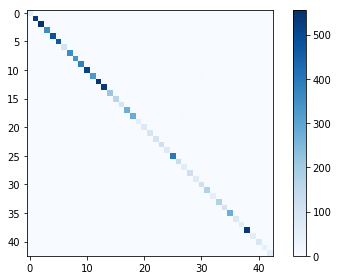The following is the log2 version of preceding screenshot: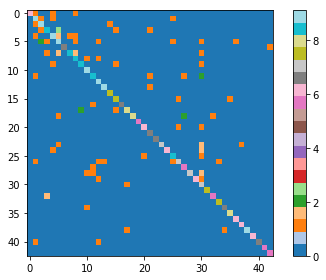# Follow-up questions

• Try adding/removing some CNN layers and/or fully connected layers. How does the performance change?
• This simple project is proof that dropouts are necessary for regularization. Change the dropout percentage and check the overfitting-underfitting in the output.
• Now, take a picture of multiple traffic signs in your city, and test the trained model in real life!

# Summary

In this chapter, we saw how to recognize traffic signs using a convolutional neural network, or CNN. In the next chapter, we'll see something more complex that can be done with CNNs.

• Abhishek Thakur

Abhishek Thakur is a data scientist. His focus is mainly on applied machine learning and deep learning, rather than theoretical aspects. He completed his master's in computer science at the University of Bonn in early 2014. Since then, he has worked in various industries, with a research focus on automatic machine learning. He likes taking part in machine learning competitions and has attained a third place in the worldwide rankings on the popular website Kaggle.

Browse publications by this author
• Alberto Boschetti

Alberto Boschetti is a data scientist with expertise in signal processing and statistics. He holds a Ph.D. in telecommunication engineering and currently lives and works in London. In his work projects, he faces challenges ranging from natural language processing (NLP) and behavioral analysis to machine learning and distributed processing. He is very passionate about his job and always tries to stay updated about the latest developments in data science technologies, attending meet-ups, conferences, and other events.

Browse publications by this author
• Luca Massaron

Luca Massaron is a data scientist with more than a decade of experience in transforming data into smarter artifacts, solving real-world problems, and generating value for businesses and stakeholders. He is the author of bestselling books on AI, machine learning, and algorithms. Luca is also a Kaggle Grandmaster who reached no. 7 in the worldwide user rankings for his performance in data science competitions, and a Google Developer Expert (GDE) in machine learning. He was part of books like The Kaggle Book, Machine Learning For Dummies, Algorithms for Dummies, Artificial Intelligence For Dummies. My warmest thanks go to my family, Yukiko and Amelia, for their support and loving patience.

Browse publications by this author
• Alexey Grigorev

Alexey Grigorev is a skilled data scientist, machine learning engineer, and software developer with more than 8 years of professional experience. He started his career as a Java developer working at a number of large and small companies, but after a while he switched to data science. Right now, Alexey works as a data scientist at Simplaex, where, in his day-to-day job, he actively uses Java and Python for data cleaning, data analysis, and modeling. His areas of expertise are machine learning and text mining.

Browse publications by this author
• Rajalingappaa Shanmugamani

Rajalingappaa Shanmugamani is currently working as an Engineering Manager for a Deep learning team at Kairos. Previously, he worked as a Senior Machine Learning Developer at SAP, Singapore and worked at various startups in developing machine learning products. He has a Masters from Indian Institute of TechnologyMadras. He has published articles in peer-reviewed journals and conferences and submitted applications for several patents in the area of machine learning. In his spare time, he coaches programming and machine learning to school students and engineers.

Browse publications by this author
Latest Reviews (2 reviews total)
It was a great value and I look forward to learning all of the techniques in the books.
Anlatım ve örnekler çok iyi. Kodların hepsi çalıştırılabiliyor.